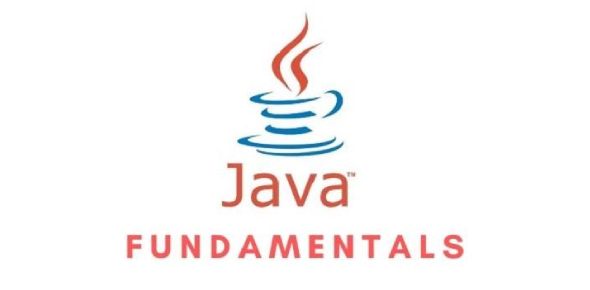# Java Fundamentals Test

30 Questions | Total Attempts: 1806SettingsHi

Related Topics
• 1.
Choose the operations that can be performed on String objects: (A) + (B) + = (C) - (D) % (E) ^
• A.

(A)

• B.

(D)

• C.

(A) and (B)

• D.

(D) and (E)

• E.

None of the above

• 2.
Which three are valid declarations of a float? 1.    float f1 = -343; 2.    float f2 = 3.14; 3.    float f3 = 0x12345; 4.    float f4 = 42e7; 5.    float f5 = 2001.0D; float f6 = 2.81F;
• A.

1,2,4

• B.

1,3,6

• C.

2,3,4

• D.

2,5,6

• 3.
What is the numerical range of a char?
• A.

-128 to 127

• B.

-232 to -231

• C.

0 to 32767

• D.

0 to 65535

• 4.
Which one of these lists contains only Java programming language keywords?
• A.

Class, if, void, long, Int, continue

• B.

Goto, instanceof, native, finally, default, throws

• C.

Try, virtual, throw, final, volatile, transient

• D.

Strictfp, constant, super, implements, do

• E.

Byte, break, assert, switch, include

• 5.
Which is a valid keyword in java?
• A.

Interface

• B.

String

• C.

Float

• D.

Unsigned

• 6.
Which is a reserved word in the Java programming language?
• A.

Method

• B.

Native

• C.

Subclasses

• D.

Reference

• E.

Array

• 7.
• A.

Char c1 = 064770;

• B.

Char c2 = 'face';

• C.

Char c3 = 0xbeef;

• D.

Char c4 = \u0022;

• E.

Char c5 = '\iface';

• F.

Char c6 = '\uface';

• 8.
• A.

-2147483648 and 1

• B.

0x80000000 and 0x00000001

• C.

-2147483648 and -1

• D.

1 and -2147483648

• 9.
String is a primitive datatype
• A.

True

• B.

False

• 10.
The operations y >> 3 and y >>> 3 produce the same result when y > 0.
• A.

True

• B.

False

• 11.
What will be the output of the program? class SSBool { public static void main(String [] args) { boolean b1 = true; boolean b2 = false; boolean b3 = true; if ( b1 & b2 | b2 & b3 | b2 ) /* Line 8 */ System.out.print("ok "); if ( b1 & b2 | b2 & b3 | b2 | b1 ) /*Line 10*/ System.out.println("dokey"); } }
• A.

Ok

• B.

Dokey

• C.

Ok dokey

• D.

Compilatioon error

• 12.
The expression (y >= z && a == b) is evaluated by first evaluating the expression y >= z, and then evaluating a == b.
• A.

True

• B.

False

• 13.
Which statements about the output of the following program are true? public class EqualTest { public static void main(String args[]) { String s1 = “YES”; String s2 = “YES”; if ( s1 == s2 ) System.out.println(“equal”); String s3 = new String(“YES”); String s4 = new String(“YES”); if ( s3 == s4 ) System.out.println(“s3 eq s4”); } }
• A.

“equal” is printed, “s3 eq s4” is printed.

• B.

“equal” is printed only.

• C.

“s3 eq s4” is printed only.

• D.

Nothing is printed.

• 14.
What is the difference between >> and >>>?
• A.

The >> operator shifts left and the >>> operator shifts right.

• B.

The >> operator shifts right and the >>> operator shifts left.

• C.

The >> operator fills the shifted out high order bits based on the sign bit and the >>> operator fills the high order bits with zeros.

• D.

The >>> operator fills the shifted out high order bits based on the sign bit and the >>> operator fills the high order bits with zeros.

• 15.
Null is a keyword in java
• A.

True

• B.

False

• 16.
Which of the following are Java modifiers?
• A.

Public

• B.

Private

• C.

Transient

• D.

Friendly

• E.

Vagrant

• 17.
Given the following variables which of the following lines will compile without error? String s = "Hello"; long l = 99; double d = 1.11; int i = 1; int j = 0; Choose 2
• A.

J=i

• B.

J=i

• C.

J=i

• D.

J=i

• 18.
What will be output by the following line? System.out.println(Math.floor(-2.1));
• A.

-2

• B.

2.0

• C.

-3

• D.

-3.0

• 19.
What will be output by the following line of code? System.out.println(010|4);
• A.

14

• B.

0

• C.

6

• D.

12

• 20.
Which of the following has highest operator precedence
• A.

++

• B.

?:

• C.

• D.

&&

• E.

=

• 21.
Given the following variables char c = 'c'; int i = 10; double d = 10; long l = 1; String s = "Hello"; Which of the following will compile without error?
• A.

C=c+i;

• B.

S+=i;

• C.

I+=s;

• D.

C+=s;

• 22.
• A.

True

• B.

False

• 23.
Default value of the bolean is false
• A.

True

• B.

False

• 24.
Extended assignment operators (for example +=) do an implicit cast. byte b = 10; b += 10;
• A.

True

• B.

False

• 25.
Which of the following are legal identifiers. Choose all that apply
• A.

2variable

• B.

Variable2

• C.

_whatavariable

• D.

_3_

• E.

\$anothervar

• F.

#myvar